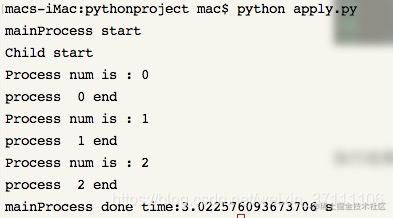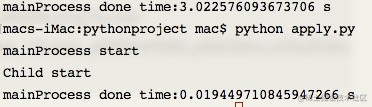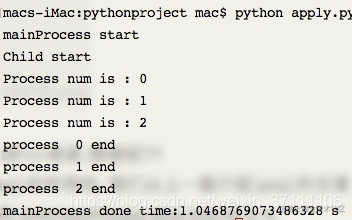# 参考网址

https://blog.csdn.net/weixin_37111106/article/details/85122988

# 1/二者的区别

``````apply():
阻塞主进程，非异步(子进程不是同时执行的)
它的非异步体现在：一个一个按顺序地执行子进程,
它的堵塞体现在：等到全部子进程都执行完毕后，继续执行apply()后面主进程的代码。

apply_async()：
非阻塞主进程，异步。
它的非堵塞体现在：他不会等待子进程完全执行完毕, 主进程会继续执行, 他会根据系统调度来进行进程之间的切换。如果想堵塞主要进程，需要用.join()函数来堵塞主进程。
它的异步体现在：子进程之间是同时执行的。
``````

# 2/apply()

```python import time import multiprocessing

def doIt(num): print("Process num is : %s" % num) time.sleep(1) print('process %s end' % num)

if name == 'main': print('mainProcess start') #记录一下开始执行的时间 start_time = time.time() #创建三个子进程 pool = multiprocessing.Pool(3) print('Child start') for i in range(3): pool.apply(doIt,[i]) print('mainProcess done time:%s s' % (time.time() - start_time))

```

``````结果如下所示：

``````# 3/apply_async()

``````接下来是使用apply_async(), 只需要把上面的代码使用 apply()的地方改成apply_async() 即可, 代码不再贴上

````````````那么我们在使用apply_async()函数是不是就不能执行子进程呢？肯定可以啊！！！小老弟，想啥呢？？

join()就是告诉主进程老子要运行子进程了，你先等等。
``````

```python import time import multiprocessing

def doIt(num): print("Process num is : %s" % num) time.sleep(1) print('process %s end' % num)

if name == 'main': print('mainProcess start') #记录一下开始执行的时间 start_time = time.time() #创建三个子进程 pool = multiprocessing.Pool(3) print('Child start') for i in range(3): pool.apply_async(doIt,[i])

``````pool.close()
pool.join()

print('mainProcess done time:%s s' % (time.time() - start_time))
``````

```

``````结果如下所示：

CPU在执行第一个子进程的时候, 还没等第一个子进程结束, 系统调度到了按顺序调度到了第二个子进程,

``````